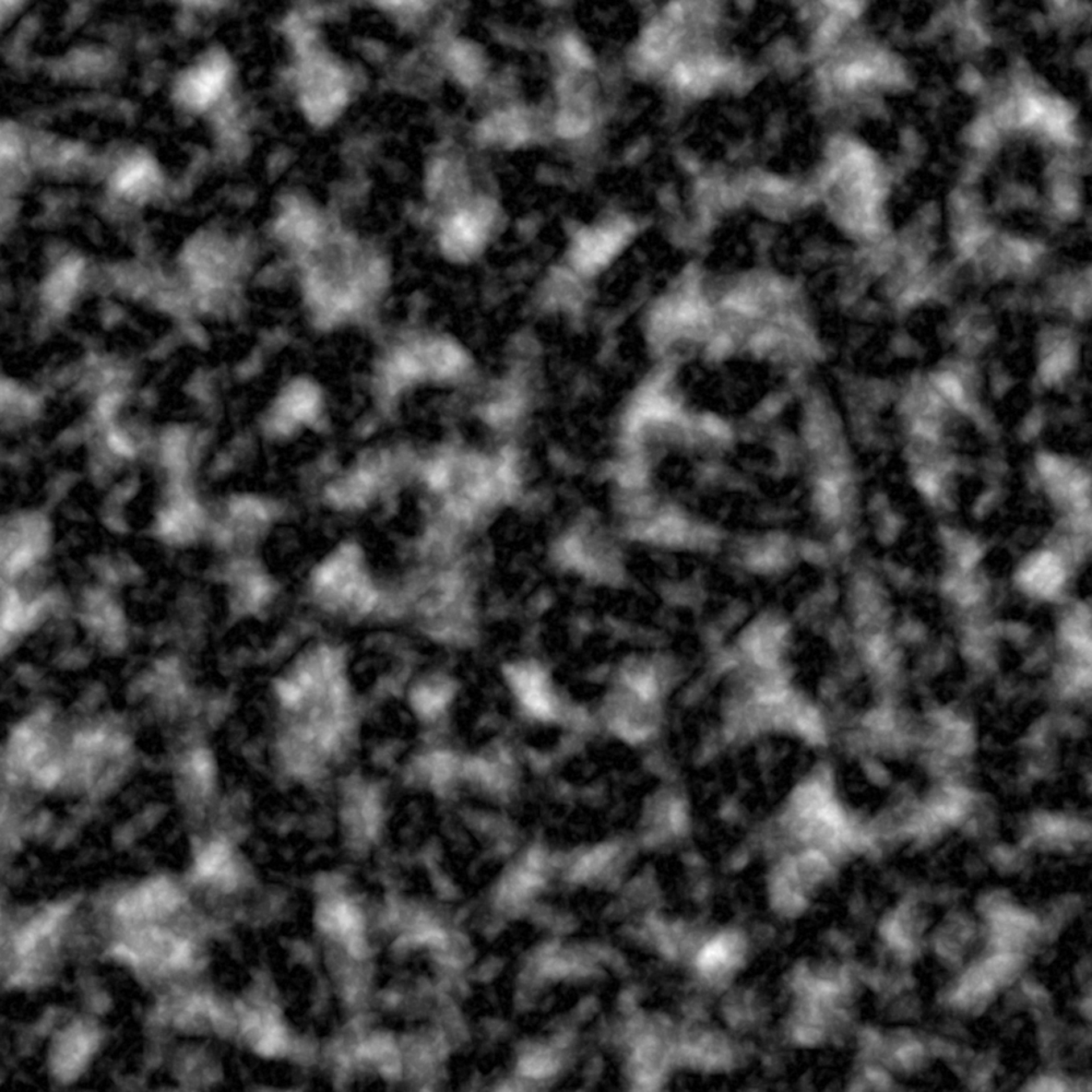This fractal is a slight variation of fbm() fractal. Before adding the new octave to the cumulated values it will clamp it between a minimum and maximum value. This function is intended to be used in conjunction with fracture()

clamped(base, new, strength, min = 0, max = Inf, ...)

## Arguments

base The prior values to modify The new values to modify base with A value to modify new with before applying it to base The upper and lower bounds of the noise values ignored

Other Fractal functions: billow(), fbm(), ridged()
grid <- long_grid(seq(1, 10, length.out = 1000), seq(1, 10, length.out = 1000))
grid$simplex <- fracture(gen_simplex, clamped, octaves = 8, x = grid$x,